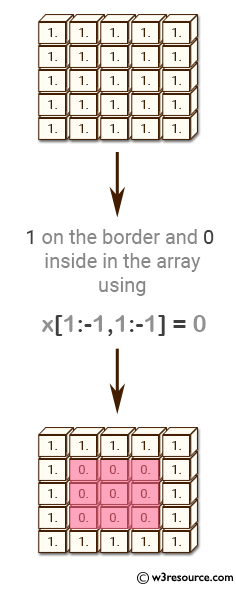﻿ NumPy: Create a 2d array with 1 on the border and 0 inside - w3resource# NumPy: Create a 2d array with 1 on the border and 0 inside

## NumPy: Array Object Exercise-8 with Solution

Write a NumPy program to create a 2d array with 1 on the border and 0 inside.Sample Solution:-

Python Code:

``````import numpy as np
x = np.ones((5,5))
print("Original array:")
print(x)
print("1 on the border and 0 inside in the array")
x[1:-1,1:-1] = 0
print(x)
```
```

Sample Output:

```Original array:
[[ 1.  1.  1.  1.  1.]
[ 1.  1.  1.  1.  1.]
[ 1.  1.  1.  1.  1.]
[ 1.  1.  1.  1.  1.]
[ 1.  1.  1.  1.  1.]]
1 on the border and 0 inside in the array
[[ 1.  1.  1.  1.  1.]
[ 1.  0.  0.  0.  1.]
[ 1.  0.  0.  0.  1.]
[ 1.  0.  0.  0.  1.]
[ 1.  1.  1.  1.  1.]]
```

Python Code Editor:

Have another way to solve this solution? Contribute your code (and comments) through Disqus.

What is the difficulty level of this exercise?

Test your Python skills with w3resource's quiz

﻿

## Python: Tips of the Day

While-else construct:

```i = 5

while i > 1:
print("Whil-ing away!")
i -= 1
if i == 3:
break
else:
print("Finished up!")
```